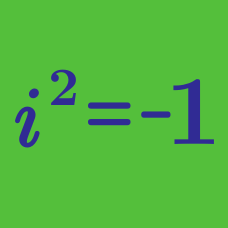Algebra

# Complex Numbers Warmup

True or False:

For complex numbers $a$ and $b$,

$|a| \times |b| = | a \times b |.$

Evaluate

$i \times ( -i) .$

Note: $i$ is the imaginary unit, where $i^2=-1$.

For any complex number $z$, which of the following must be equal to

"the real part of the imaginary part of $z$"?

Which of the following is equal to

$\frac{ 1 + i } { 1 - i }?$

Are real numbers also complex numbers?

×